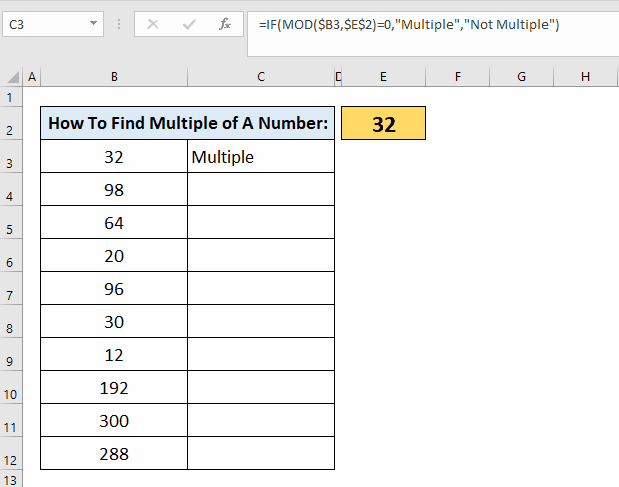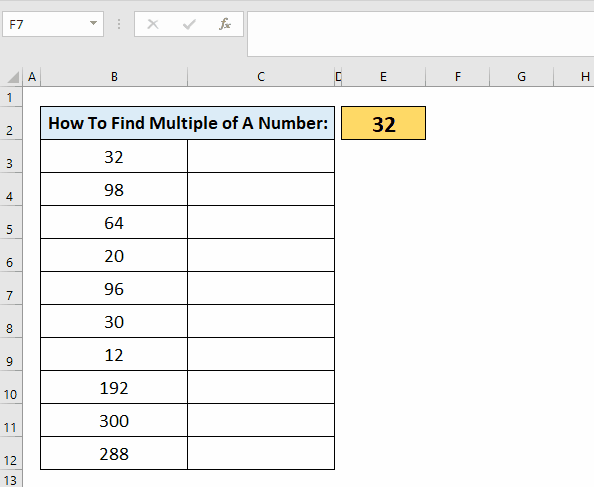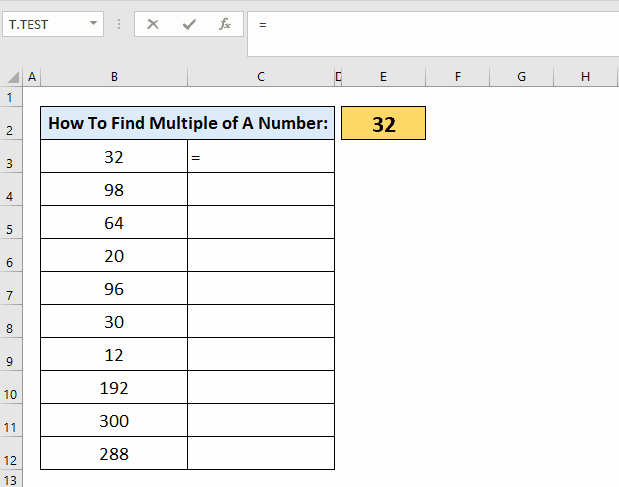# How To Check If A Number Is A Multiple Of Another

## Understanding the Concept of Multiples

Before diving into the practical aspects of checking for multiples in Excel, it is essential to grasp the concept of multiples. In mathematics, a multiple of a given number is obtained by multiplying that number by an integer. For example, in the case of the number 4, its multiples include 4, 8, 12, 16, and so on. Essentially, multiples are the numbers that can be evenly divided by a specific number without leaving a remainder. By utilizing Excel’s functionalities, you can efficiently determine whether a number is a multiple of another, unlocking new possibilities for data analysis and decision-making.

Here is the dataset for today’s article. We would like to find out from the available list which numbers are the multiples of the number 32.When working with numbers in Excel, it is often necessary to determine if one number is a multiple of another. Whether you’re analyzing financial data, tracking inventory, or conducting scientific research, the ability to check for multiples can provide valuable insights. Fortunately, Excel offers a straightforward and efficient method to perform this task.
In this article, we will guide you through the process of checking if a number is a multiple of another in Excel, empowering you to make data-driven decisions with ease and confidence.

### Step 1 – Select A Cell– Select the cell where you want to apply the formula & place equals to the (=) sign.

### Step 2 – Type The Formula– Now type the formula =IF(MOD(\$B3,\$E\$2)=0, “Multiple”, “Not Multiple”).
– Press the enter button.

### Step 3 – Drag The Formula Downwards– With the help of the selection handle, drag the formula downwards.
– You can find multiple of any number with the help of the above formula.